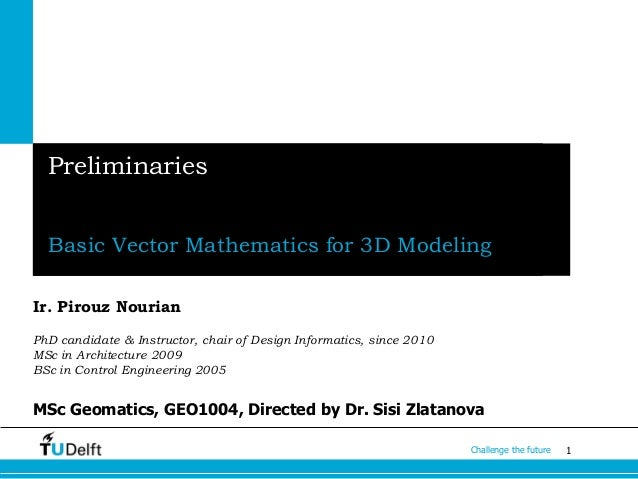Successfully reported this slideshow.Upcoming SlideShare
×

# Preliminaries of Analytic Geometry and Linear Algebra 3D modelling

1,056 views

Published on

from my lecture notes for the course Geo1004 (2015), 3D modelling of the built environment, at TU Delft, faculty of Architecture and the Built Environment

Published in: Engineering
• Full Name
Comment goes here.

Are you sure you want to Yes No• On slide number 9, there is a mistake: the term (AzBx-AxBz) should have a negative sign instead of a positive sign. Will replace the slides asap.

Are you sure you want to  Yes  No

### Preliminaries of Analytic Geometry and Linear Algebra 3D modelling

1. 1. 1Challenge the future Preliminaries Basic Vector Mathematics for 3D Modeling Ir. Pirouz Nourian PhD candidate & Instructor, chair of Design Informatics, since 2010 MSc in Architecture 2009 BSc in Control Engineering 2005 MSc Geomatics, GEO1004, Directed by Dr. Sisi Zlatanova
2. 2. 2Challenge the future INVISIBLE DIRECTIONS Vector Mathematics in a Nutshell René Descartes Image courtesy of David Rutten, from Rhinoscript 101
3. 3. 3Challenge the future INVISIBLE DIRECTIONS Basic Operations 𝐴 = 𝑎 𝑥 𝒊 + 𝑎 𝑦 𝒋 + 𝑎 𝑧 𝒌 𝐵 = 𝑏 𝑥 𝒊 + 𝑏 𝑦 𝒋 + 𝑏 𝑧 𝒌 𝐴 + 𝐵 = (𝑎 𝑥 + 𝑏 𝑥)𝒊 + (𝑎 𝑦+𝑏 𝑦)𝒋 + (𝑎 𝑧+𝑏 𝑧)𝒌 Vector Addition Vector Length 𝐴 = 𝑎 𝑥 2 + 𝑎 𝑦 2 + 𝑎 𝑧 2
4. 4. 4Challenge the future Dot Product: physical intuition… E.g. How to detect perpendicularity? • Image courtesy of http://sdsu-physics.org
5. 5. 5Challenge the future Dot Product: How is it calculated in analytic geometry? Image courtesy of http://sdsu- 𝜃 B A 𝒊. 𝒊 = 𝒋. 𝒋 = 𝒌. 𝒌 = 1 𝒊. 𝒋 = 𝒋. 𝒊 = 0 𝒋. 𝒌 = 𝒌. 𝒋 = 0 𝒌. 𝒊 = 𝒊. 𝒌 = 0
6. 6. 6Challenge the future Dot Product: How is it calculated in analytic geometry? 𝐴 = 𝑎 𝑥 𝒊 + 𝑎 𝑦 𝒋 + 𝑎 𝑧 𝒌 = 𝑎 𝑥 𝑎 𝑦 𝑎 𝑧 𝒊 𝒋 𝒌 𝐵 = 𝑏 𝑥 𝒊 + 𝑏 𝑦 𝒋 + 𝑏 𝑧 𝒌 = 𝑏 𝑥 𝑏 𝑦 𝑏 𝑧 𝒊 𝒋 𝒌 𝐴. 𝐵 == 𝐴 . 𝐵 . 𝐶𝑜𝑠(𝜃) 𝜃 B A 𝐴. 𝐵 = 𝑎 𝑥 𝑎 𝑦 𝑎 𝑧 𝑏 𝑥 𝑏 𝑦 𝑏 𝑧 = 𝑎 𝑥 𝑏 𝑥 + 𝑎 𝑦 𝑏 𝑦 + 𝑎 𝑧 𝑏 𝑧
7. 7. 7Challenge the future Cross Product: physical intuition… • Image courtesy of http://hyperphysics.phy-astr.gsu.edu Images courtesy of Raja Issa, Essential Mathematics for Computational Design E.g. How to detect parallelism?
8. 8. 8Challenge the future Cross Product: How is it calculated in analytic geometry? Images courtesy of Raja Issa, Essential Mathematics for Computational Design 𝒊 × 𝒊 = 𝒋 × 𝒋 = 𝒌 × 𝒌 = 𝟎 𝒊 × 𝒋 = 𝒌 𝒋 × 𝒌 = 𝒊 𝒌 × 𝒊 = 𝒋 𝒋 × 𝒊 = −𝒌 𝒌 × 𝒋 = −𝒊 𝒊 × 𝒌 = −𝒋
9. 9. 9Challenge the future Cross Product: How is it calculated in analytic geometry? Images courtesy of Raja Issa, Essential Mathematics for Computational Design 𝐴 = 𝑎 𝑥 𝒊 + 𝑎 𝑦 𝒋 + 𝑎 𝑧 𝒌 = 𝑎 𝑥 𝑎 𝑦 𝑎 𝑧 𝒊 𝒋 𝒌 𝐵 = 𝑏 𝑥 𝒊 + 𝑏 𝑦 𝒋 + 𝑏 𝑧 𝒌 = 𝑏 𝑥 𝑏 𝑦 𝑏 𝑧 𝒊 𝒋 𝒌 𝐴 × 𝐵 = (𝑎 𝑥 𝒊 + 𝑎 𝑦 𝒋 + 𝑎 𝑧 𝒌) × (𝑏 𝑥 𝒊 + 𝑏 𝑦 𝒋 + 𝑏 𝑧 𝒌) = 𝒊 𝒋 𝒌 𝑎 𝑥 𝑎 𝑦 𝑎 𝑧 𝑏 𝑥 𝑏 𝑦 𝑏 𝑧 𝐴 × 𝐵 = 𝐴 . 𝐵 . 𝑆𝑖𝑛(𝜃) 𝐴 × 𝐵 = 𝑎 𝑦 𝑏 𝑧 − 𝑎 𝑧 𝑏 𝑦 𝒊 + 𝑎 𝑧 𝑏 𝑥 − 𝑎 𝑥 𝑏 𝑧 𝒋 + 𝑎 𝑥 𝑏 𝑦 − 𝑎 𝑦 𝑏 𝑥 𝒌
10. 10. 10Challenge the future INVISIBLE ORIENTATIONS Place things on planes! Planes in a Nutshell! Images courtesy of David Rutten, Rhino Script 101
11. 11. 11Challenge the future Matrix Operations [Linear Algebra]: Look these up: • Trivial Facts • Identity Matrix • Multiplication of Matrices 𝐴𝐵 ≠ 𝐵𝐴 • Transposed Matrix (𝐴 𝑇 ) 𝑇 = 𝐴 • Systems of Linear Equations • Determinant • Inverse Matrix • PCA: Eigenvalues & Eigenvectors Use MetaNumerics.DLL 𝐴𝐵𝑖,𝑗 𝑅×𝐶 = 𝐴 𝑖,𝑘 × 𝐵 𝑘,𝑗 𝑚 𝑘=1 𝐴 𝑅×𝑀 ∗ 𝐵 𝑀×𝐶 = 𝐴𝐵𝑖,𝑗 𝑅×𝐶
12. 12. 12Challenge the future TRANSFORMATIONS • Linear Transformations: Euclidean and Affine • Homogenous Coordinate System • Inverse Transforms? • Non-Linear Transformations? Images courtesy of Raja Issa, Essential Mathematics for Computational Design 𝐿𝑖𝑛𝑒𝑎𝑟 𝑇𝑟𝑎𝑛𝑠𝑓𝑜𝑟𝑚𝑎𝑡𝑖𝑜𝑛𝑠 by Matrices
13. 13. 13Challenge the future TOPOLOGY in GH: Use matrices to represent graphs Connectivity, Adjacency and Graphs in GH We will see more about topology in solids and meshes!
14. 14. 14Challenge the future Questions?